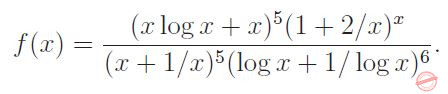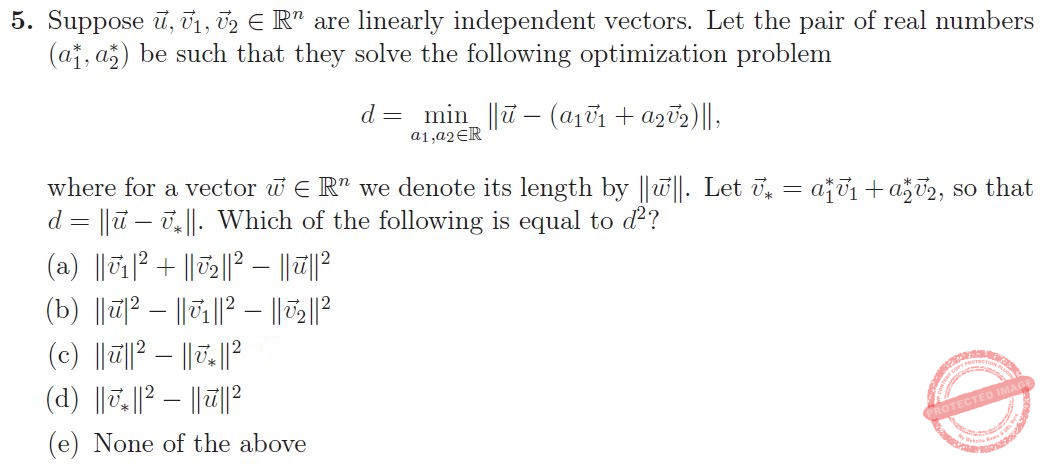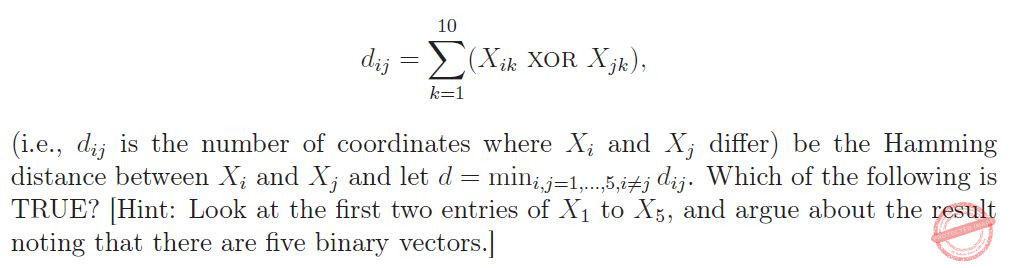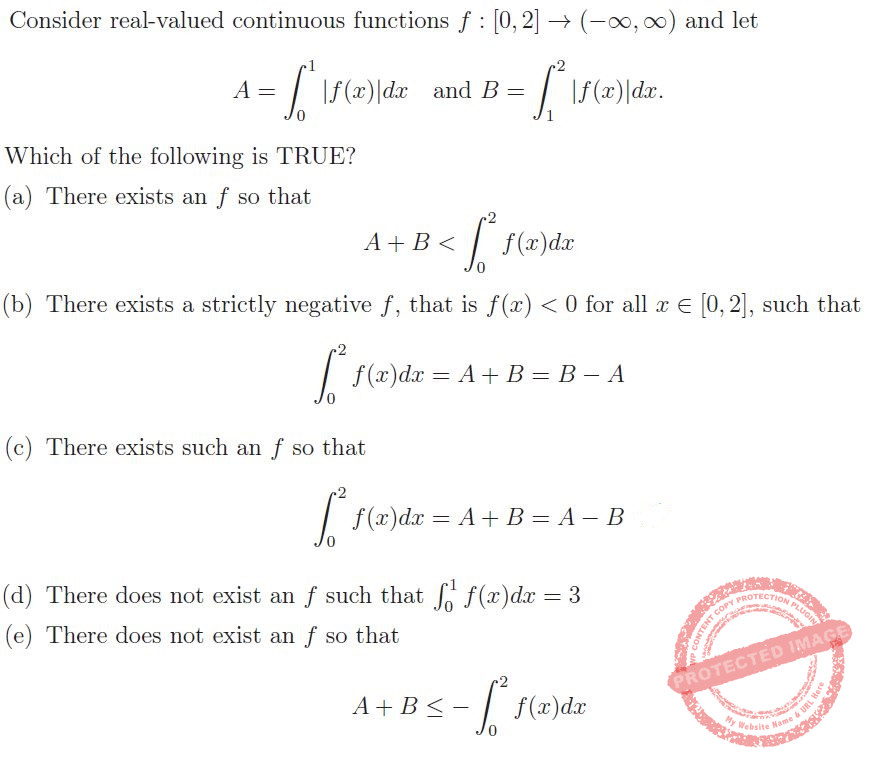## TIFR PHD CS & SS 2018

 Question 1
Consider a point A inside a circle C that is at distance 9 from the centre of the circle. Suppose you are told that there is a chord of length 24 passing through A with A as its midpoint. How many distinct chords of C have integer length and pass through
 A 2 B 6 C 7 D 12 E 14
Engineering-Mathematics       Co-ordinate-Geometry
 Question 2
Consider the following subsets of R3 (the first two are cylinders, the third is a plane):
C1 = {(x, y, z) : y2 + z2 ≤ 1};
C2 = {(x, y, z) : x2 + z2 ≤ 1};
H = {(x, y, z) : z = 0.2}.
Let A = C1 ∩ C2 ∩ H. Which of the following best describes the shape of the set A?
 A Circle B Ellipse C Triangle D Square E An octagonal convex figure with curved sides
Engineering-Mathematics       Set-Theory
 Question 3
Which of the following statements is TRUE for all sufficiently large integers n?
 A 22√(log log n) < 2 < √(log n) < n B 2√(log n) < n < 22√(log log n) C n < 2√(log n) < 22√(log log n) D n < 22√(log log n) < 2√(log n) < E 2√(log n) < 22√(log log n) < n
Engineering-Mathematics       Logarithms
 Question 4
The distance from your home to your office is 4 kilometres and your normal walking speed is 4 km/hr. On the first day, you walk at your normal walking speed and take time T1 to reach office.
On the second day, you walk at a speed of 3 km/hr for 2 kilometres, and at a speed of 5 km/hr for the remaining 2 kilometres and you take time T2 to reach office.
On the third day, you walk at a speed of 3 km/hr for 30 minutes, and at 5 km/hr for the remaining time and take time T3 to reach office.
What can you say about the ordering of T1, T2 and T3?
 A T1 > T2 and T1 < T3. B T1 = T2 = T3 C T1 < T2 and T1 < T3 D T1 = T2 and T1 < T3 E T1 < T2 and T1 = T3
Aptitude       Numerical
 Question 5
Which of the following is the derivative of f(x) = xx when x > 0?
 A xx B xx In x C xx + xx In x D (xx)(xxIn x) E None of the above; function is not differentiable for x > 0
Engineering-Mathematics       Calculus
 Question 6
What is the minimum number of students needed in a class to guarantee that there are at least 6 students whose birthdays fall in the same month?
 A 6 B 23 C 61 D 72 E 91
Engineering-Mathematics       Combinatorics
 Question 7
Consider the following function definition.
void greet (int n)
{
if (n>0)
{
printf("hello");
greet(n-1);
}
printf("world");
}
If you run greet(n) for some non-negative integer n, what would it print?
 A n times “hello”, followed by n + 1 times “world” B n times “hello”, followed by n times “world” C n times “helloworld” D n + 1 times “helloworld” E n times “helloworld”, followed by “world”
Programming       Functions
 Question 8
A crime has been committed with four people at the scene of the crime. You are responsible for finding out who did it. You have recorded the following statements from the four witnesses, and you know one of them has committed the crime.
(1) Anuj says that Binky did it.
(2) Binky says that Anuj did it.
(3) Chacko says that Binky is telling the truth.
(4) Desmond says that Chacko is not lying.
You know that exactly three of the statements recorded are FALSE. Who committed the crime?
 A Anuj B Binky C Chacko D Desmond E Either Anuj or Binky; the information is insufficient to pinpoint the criminal
Logical-Reasoning       Logical-Reasoning
 Question 9
How many ways are there to assign colours from the range {1, 2, . . . , r} to the vertices of the following graph so that adjacent vertices receive distinct colours?A r4 B r4-4r3 C r4-5r3+8r2-4r D r4-4r3+9r2-3r E r4-5r3+10r2-15r
Engineering-Mathematics       Graph-Theory
 Question 10
Let C be a biased coin such that the probability of a head turning up is p. Let pn denote the probability that an odd number of heads occurs after n tosses for n∈{0,1,2, . . . }. Then, which of the following is TRUE?
 A pn = 1/2 for all n ∈ {0, 1, 2, . . . }. B pn =(1-p)(1-pn-1+p.pn-1 for n≥1 and p0=0 C pn =Σni-1(1-2p)I-1 for n ≥ 1. D If p = 1/2, then pn = 1/2 for all n ∈ {0, 1, 2, . . . }. E pn = 1 if n is odd and 0 otherwise.
Engineering-Mathematics       Probability-and-statistics
 Question 11
We are given a (possibly empty) set of objects. Each object in the set is colored either black or white; is shaped either circular or rectangular, and has a profile that is either fat or thin. These properties obey the following principles:
1. Each white object is also circular.
2. Not all thin objects are black.
3. Each rectangular object is also either thin or white or both thin and white.
Consider the following statements:
(i) If there is a thin object in the set, then there is also a white object.
(ii) If there is a rectangular object in the set, then there are at least two objects.
(iii) Every fat object in the set is circular.
Which of the above statements must be TRUE for the set?
 A (i) only B (i) and (ii) only C (i) and (iii) only D None of the statements must be TRUE E All of the statements must be TRUE
Reasoning       Logical-Reasoning
 Question 12
An n × n matrix M with real entries is said to be positive definite if for every nonzero n-dimensional vector x with real entries, we have xTMx > 0. Let A and B be symmetric, positive definite matrices of size n × n with real entries. Consider the following matrices, where I denotes the n × n identity matrix:
(1) A + B
(2) ABA
(3) A2 + I
Which of the above matrices must be positive definite?
 A Only (2) B Only (3) C Only (1) and (3) D None of the above matrices are positive definite E All of the above matrices are positive definite
Engineering-Mathematics       Linear-Algebra
 Question 13
A hacker knows that the password to the TIFR server is a 10-letter string consisting of lower-case letters from the English alphabet. He guesses a set of 5 distinct 10-letter strings (with lower-case letters) uniformly at random. What is the probability that one of the 5 guesses of the hacker is the correct password?A a only B b only C c only D d only E e only
Engineering-Mathematics       Probability-and-statistics
 Question 14
Let A be an n × n invertible matrix with real entries whose row sums are all equal to c. Consider the following statements:
(1) Every row in the matrix 2A sums to 2c.
(2) Every row in the matrix A2 sums to c2.
(3) Every row in the matrix A−1 sums to c−1.
Which of the following is TRUE?
 A none of the statements (1), (2), (3) is correct B statement (1) is correct but not necessarily statements (2) or (3) C statement (2) is correct but not necessarily statements (1) or (3) D statements (1) and (2) are correct but not necessarily statement (3) E all the three statements (1), (2), and (3) are correct
Engineering-Mathematics       Linear-Algebra
 Question 15
Suppose a box contains 20 balls: each ball has a distinct number in {1, . . . , 20} written on it. We pick 10 balls (without replacement) uniformly at random and throw them out of the box. Then we check if the ball with number “1” on it is present in the box. If it is present, then we throw it out of the box; else we pick a ball from the box uniformly at random and throw it out of the box.
What is the probability that the ball with number “2” on it is present in the box?
 A 9/20 B 9/19 C 1/2 D 10/19 E None of the above
Engineering-Mathematics       Probability-and-statistics
 Question 16
What is the remainder when 44444444 is divided by 9?
 A 1 B 2 C 5 D 7 E 8
Engineering-Mathematics       Combinatorics
 Question 17
Consider the following non-deterministic automaton, where s1 is the start state and s4 is the final (accepting) state. The alphabet is {a, b}. A transition with label  can be taken without consuming any symbol from the input.A (a + b)∗aba B aba(a + b)∗aba C (a + b)aba(b + a)∗ D aba(a + b)∗ E (ab)∗aba
Theory-of-Computation       Regular-Expression
 Question 18
How many distinct minimum weight spanning trees does the following undirected, weighted graph have?A 1 B 2 C 4 D 6 E 8
Algorithms       Minimum-Spanning-Tree
 Question 19
The notation “⇒” denotes “implies” and “∧” denotes “and” in the following formulae.
Let X denote the formula: (b ⇒ a) ⇒ (a ⇒ b)
Let Y denote the formula: (a ⇒ b) ∧ b
Which of the following is TRUE?
 A X is satisfiable and Y is not satisfiable. B X is satisfiable and Y is a tautology. C X is not a tautology and Y is not satisfiable. D X is not a tautology and Y is satisfiable. E X is a tautology and Y is satisfiable.
Engineering-Mathematics       Propositional-Logic
 Question 20
Which of the following functions, given by their recurrences, grows the fastest asymptotically?
 A T(n) = 4 · T(n/2) + 10n B T(n) = 8 · T(n/3) + 24n2 C T(n) = 16 · T(n/4) + 10n2 D T(n) = 25 · T(n/5) + 20(n log n)1.99 E They are all asymptotically the same.
Algorithms       Asymptotic-Complexity
 Question 21
Consider the following implementation of a binary tree data structure. The operator + denotes list-concatenation. That is, [a,b,c] + [d,e] = [a,b,c,d,e].
struct TreeNode:
int value
TreeNode leftChild
TreeNode rightChild
function preOrder(T):
if T == null:
return []
else:
return [T.value] + preOrder(T.leftChild) + preOrder(T.rightChild)
function inOrder(T):
if T == null:
return []
else:
return inOrder(T.leftChild) + [T.value] + inOrder(T.rightChild)
function postOrder(T):
if T == null:
return []
else:
return postOrder(T.leftChild) + postOrder(T.rightChild) + [T.value]
For some T the functions inOrder(T) and preOrder(T) return the following:
inOrder(T): [12,10,6,9,7,2,15,5,1,13,4,3,8,14,11]
preOrder(T): [5,2,10,12,9,6,7,15,13,1,3,4,14,8,11]
What does postOrder(T) return?
 A [12,6,10,7,15,2,9,1,4,13,8,11,14,3,5] B [11,8,14,4,3,1,13,15,7,6,9,12,10,2,5] C [11,14,8,3,4,13,1,5,15,2,7,9,6,10,12] D [12,6,7,9,10,15,2,1,4,8,11,14,3,13,5] E Cannot be uniquely determined from given information.
Data-Structures       Binary-Trees
 Question 22
Consider the recursive quicksort algorithm with “random pivoting”. That is, in each recursive call, a pivot is chosen uniformly at random from the sub-array being sorted. When this randomized algorithm is applied to an array of size n all whose elements are distinct, what is the probability that the smallest and the largest elements in the array are compared during a run of the algorithm?
 A 1/n B 2/n C Θ(1/n log n) D O(1/n2) E Θ(1/n log2 n)
Algorithms       Algorithms Sorting
 Question 23
In an undirected graph G with n vertices, vertex 1 has degree 1, while each vertex 2, . . ., n − 1 has degree 10 and the degree of vertex n is unknown. Which of the following statements must be TRUE of the graph G?
 A There is a path from vertex 1 to vertex n B There is a path from vertex 1 to each vertex 2, . . ., n − 1 C Vertex n has degree 1 D The diameter of the graph is at most n/10 E All of the above choices must be TRUE
Engineering-Mathematics       Graph-Theory
 Question 24
Let G = (V,E) be a DIRECTED graph, where each edge e has a positive weight w(e), and all vertices can be reached from vertex s. For each vertex v, let φ(v) be the length of the shortest path from s to v. Let G' = (V,E) be a new weighted graph with the same vertices and edges, but with the edge weight of every edge e = (u → v) changed to w'(e) = w(e) + φ(v) − φ(u).
Let P be a path from s to a vertex v, and let w(P) = ∑e∈P we, and w'(P) = ∑e∈P w'e
Which of the following options is NOT NECESSARILY TRUE?
 A If P is a shortest path in G, then P is a shortest path in G'. B If P is a shortest path in G', then P is a shortest path in G. C If P is a shortest path in G, then w'(P) = 2 × w(P). D If P is NOT a shortest path in G, then w'(P) < 2 × w(P). E All of the above options are necessarily TRUE.
Algorithms       Shortest-Path
 Question 25
For two n-bit strings x, y ∈ {0, 1}n, define z := x ⊕ y to be the bitwise XOR of the two strings (that is, if xi, yi, zi denote the i-th bits of x, y, z respectively, then zi = xi + yi mod 2). A function h:{0, 1}n →{0, 1}n is called linear if h(x⊕y) = h(x)⊕h(y), for every x,y ∈ {0, 1}n. The number of such linear functions for n ≥ 2 is:
 A 2n B 2n2 C 2(n 2) D 24n E 2n2+n
Engineering-Mathematics       Relations-and-Functions
 Question 26
Consider the language L ⊆ {a,b,c} defined as L = {apbqcr : p = q or q = r or r = p} . Which of the following answers is TRUE about the complexity of this language?
 A L is regular but not context-free. B L is context-free but not regular. C L is decidable but not context-free. D The complement of L, defined as L' = {a, b, c}∗ \ L, is regular. E L is regular, context-free and decidable.
Theory-of-Computation       Languages-and-Grammars
 Question 27
Consider the following statements:
(i) For every positive integer n, let #n be the product of all primes less than or equal to n. Then, #p + 1 is a prime, for every prime p.
(ii) π is a universal constant with value 22/7.
(iii) No polynomial time algorithm exists that can find the greatest common divisor of two integers given as input in binary.
(iv) Let L≡{x∈{0, 1} | x is the binary encoding of an integer that is divisible by 31}. Then, L is a regular language.
Then which of the following is TRUE?
 A Only statement (i) is correct. B Only statement (ii) is correct. C Only statement (iii) is correct. D Only statement (iv) is correct. E None of the statements are correct.
 Question 28
Let n ≥ 3, and let G be a simple, connected, undirected graph with the same number n of vertices and edges. Each edge of G has a distinct real weight associated with it. Let T be the minimum weight spanning tree of G. Which of the following statements
is NOT ALWAYS TRUE?
 A The minimum weight edge of G is in T. B The maximum weight edge of G is not in T. C G has a unique cycle C and the minimum weight edge of C is also in T. D G has a unique cycle C and the maximum weight edge of C is not in T. E T can be found in O(n) time from the adjacency list representation of G.
Algorithms       Minimum-Spanning-Tree
 Question 29
Define the language INFINITEDFA ≡ {A|A is a DFA and L(A) is an infinite language}, where A denotes the description of the deterministic finite automata (DFA). Then which of the following about INFINITEDFA is TRUE:
 A It is regular. B It is context-free but not regular. C It is Turing decidable (recursive). D It is Turing recognizable but not decidable. E Its complement is Turing recognizable but it is not decidable.
Theory-of-Computation       Languages-and-Grammars
 Question 30
G represents an undirected graph and a cycle refers to a simple cycle (no repeated edges or vertices). Define the following two languages.
SCYCLE = { (G, k) | G contains a cycle of length at most k}
and
LCYCLE = { (G, k) | G contains a cycle of length at least k}
Which of the following is NOT known to be TRUE (to the best of our current knowledge)?
 A SCYCLE ∈ P. B LCYCLE ∈ NP. C LCYCLE ≤P SCYCLE (i.e, there is a polynomial time many-to-one reduction from LCYCLE to SCYCLE) D LCYCLE is NP-complete. E SCYCLE ≤P LCYCLE (i.e, there is a polynomial time many-to-one reduction from SCYCLE to LCYCLE)
Algorithms       P-NP
 Question 31
Consider a discrete-time system which in response to input sequence x[n] (n integer) outputs the sequence y[n] such thatWhich of the following describes the system?
 A Linear, time-invariant B Linear, time-variant C Non-linear, time-invariant D Non-linear, time-variant E Cannot be determined from the information given
 Question 32
A hotel has n rooms numbered 1, 2, . . . , n. For each room there is one spare key labeled with the room number. The hotel manager keeps all the spare keys in a box. Her mischievous son got hold of the box and permuted the labels uniformly at random. What is the expected number of keys which still open the room whose label they carry? [Hint: Use linearity of expectation]
 A 1 B (n-1)/n C n/(n-1) D n/2 E None of the above
Engineering-Mathematics       Probability-and-statistics
 Question 33
Let limn→∞ f(n) = ∞ and limn→∞ g(n) = ∞. Then which of the following is necessarily TRUE.
 A limn→∞ |f(n) − g(n)| = ∞ B limn→∞ |f(n) − g(n)| = 0 C limn→∞ |f(n)/g(n)| = ∞ D limn→∞ |f(n)/g(n)| = 1 E None of the above
Engineering-Mathematics       Calculus
 Question 34
ConsiderWhat can we say about limx→∞ f(x)?
 A The function f(x) does not have a limit as x→∞ B limx→∞ f(x) = e2 C limx→∞ f(x) = e1/2 D limx→∞ f(x) = 0 E limx→∞ f(x) = ∞
Engineering-Mathematics       Calculus
 Question 35A a only B b only C c only D d only E e only
 Question 36
Consider the system shown below.If K >0, which of the following describes the system?
 A Stable, causal B Stable, non-causal C Unstable, non-causal D Unstable, causal E Cannot be determined from the information given
 Question 37
Let X1,X2 and X3 be independent random variables with uniform distribution over [0, θ]. Consider the following statements.
(i) E[max{X1,X2X3}] = (3/4)θ.
(ii) E[max{X1,X2}] − E[max{X3,X4}] = 0.
(iii) E[X1] = θ/2
(iv) E[max{X1,X2}] = (2/3)θ
Which of the above statements is/are TRUE?
 A Only (i) B Only (ii) C Only (iii) D Only (vi) E All of (i) – (iv)
Engineering-Mathematics       Probability-and-statistics
 Question 38
Let A be an n×n real matrix for which two distinct non-zero n-dimensional real column vectors v1, v2 satisfy the relation Av1 = Av2. Consider the following statements.
(i) At least one eigenvalue of A is zero.
(ii) A is not full rank.
(iii) Columns of A are not linearly independent.
(iv) det(A) = 0.
Which of the above statements is/are TRUE?
 A Only (i) B Only (ii) C Only (iii) D Only (vi) E All of (i) – (iv)
Engineering-Mathematics       Linear-Algebra
 Question 39
Let X and Y be two independent and identically distributed binary random variables that take values {−1, +1} each with probability 1/2. Let Z1 = max(X, Y ), and Z2 = min(X, Y ). Consider the following statements.
(i) Z1 and Z1 are uncorrelated
(ii) Z1 and Z2 are independent
(iii) P(Z1 = Z2) = 1/2
Which of the above statements is/are TRUE?
 A Only (i) B Only (ii) C Only (iii) D Both (i) and (ii), but not (iii) E All of (i), (ii) and (iii)
Engineering-Mathematics       Probability-and-statistics
 Question 40
Suppose that X1 and X2 denote the random outcomes of independent rolls of two dice each of which takes six values 1,2,3,4,5,6 with equal probability. What is the conditional expectation
E[X1| max(X1,X2) = 5]
 A 3 B 4 C 35/9 D 5/2 E 15/4
Engineering-Mathematics       Probability-and-statistics
 Question 41
Assume the following well known result: If a coin is flipped independently many times and its probability of heads (H) is p ∈ (0, 1) and probability of tails (T) is (1 − p), then the expected number of coin flips till the first time a heads is observed is 1/p.
What is the expected number of coin flips till the sequence HT, i.e., tails immediately following a heads, is observed for the first time?A a only B b only C c only D d only E e only
Engineering-Mathematics       Probability-and-statistics
 Question 42
Suppose that Amitabh Bachchan has ten coins in his pocket. 3 coins have tails on both sides. 4 coins have heads on both sides. 3 coins have heads on one side and tails on the other and both the outcomes are equally likely when that coin is flipped. In a bet with Dharmendra, Amitabh picks up a coin at random (each coin is equally likely to be picked) from these ten coins, flips it and finds that the outcome is tails.
What is the probability that the other side of this coin is heads?
 A 1/2 B 3/10 C 1/4 D 0.3 E 1/3
Engineering-Mathematics       Probability-and-statistics
 Question 43
Consider five distinct binary vectors X1, . . . , X5 each of length 10. LetA d = 10 B d = 9 C d = 8 D d < 8 E Information is not sufficient
 Question 44
Define the ℓp ball in two dimensions as the set of points (x, y) such that |x|p+|y|p ≤ 1. Which of the following is FALSE:
 A The ℓ2 ball is contained in the ℓ3 ball B The ℓ2 ball is contained in the ℓ1 ball C The ℓ3ball is contained in the ℓ4 ball D The ℓ2ball is contained in the ℓ5 ball E The ℓ1ball is contained in the ℓ3 ball
 Question 45A a only B b only C c only D d only E e only
Engineering-Mathematics       Probability-and-statistics
There are 45 questions to complete.